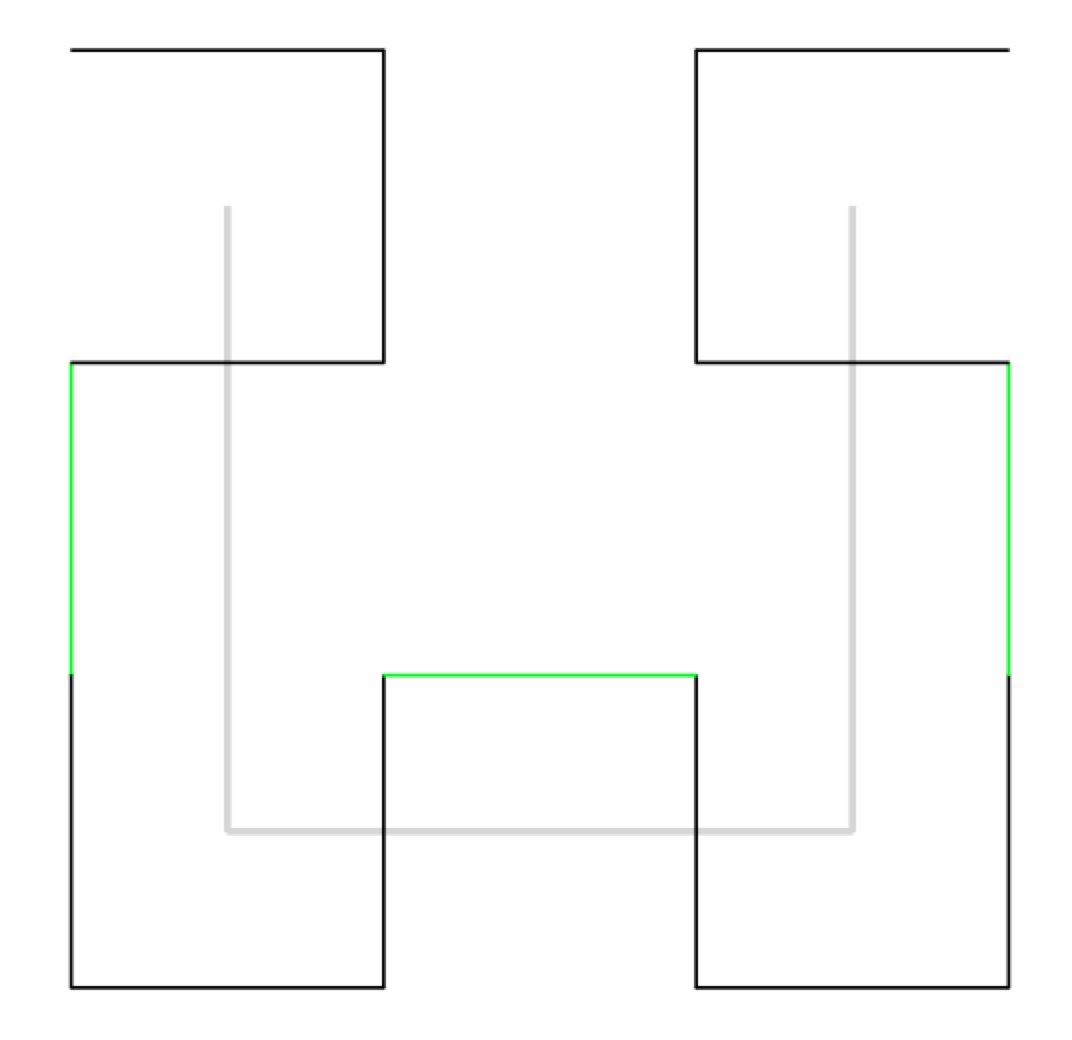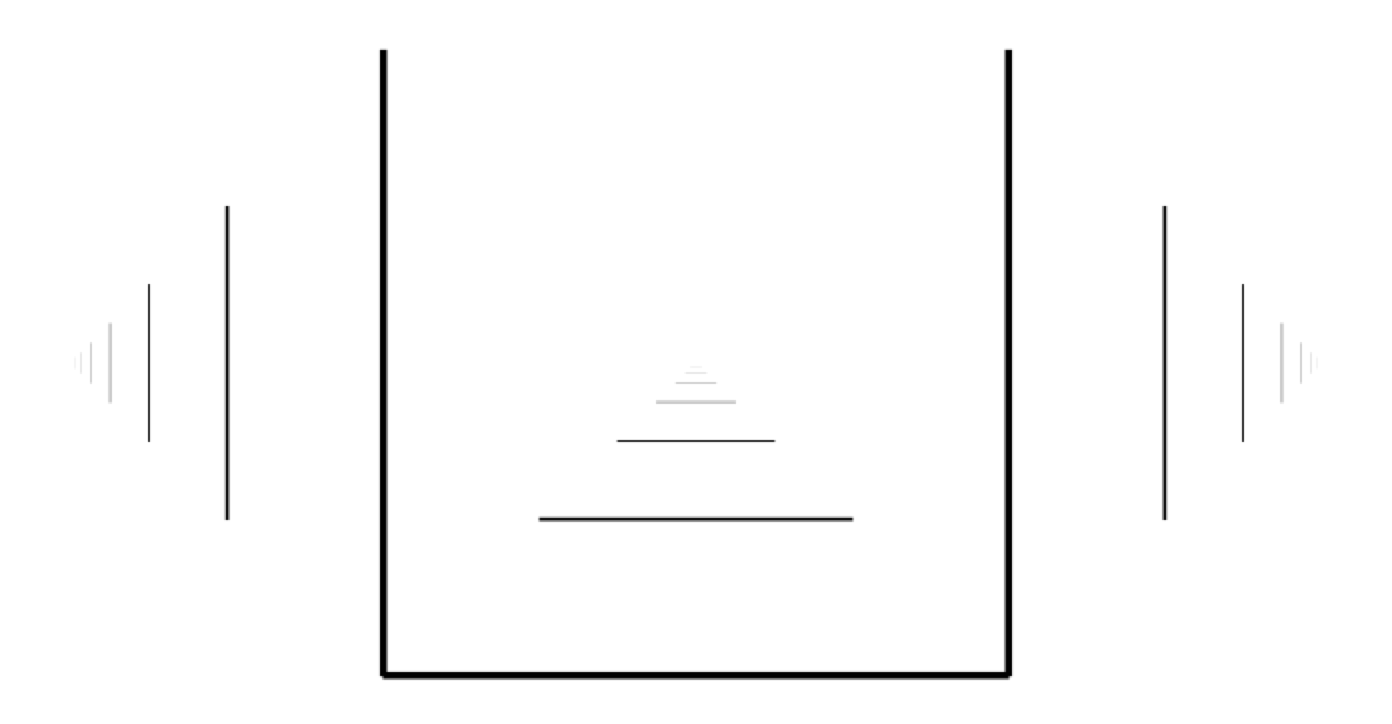## Hilbert curve in CFDG

In a previous post, I talked about creating Koch snowflakes using the context-free design grammer (CFDG) program. In this post, I'll talk about how a Hilbert curve can also be created using a trick where the basic shape we use draws part of subsequent generations for us.

Hilbert curve is a little bit trickier (it was an xkcd comic that first introduced me to this fractal) than a Koch snowflake. The first generation looks like:And the second generation looks like (I've included the first generation in gray on top of it):The lines in green connect four copies of the first generation shape together. When we go to the third generation we can see four copies of the second generation connected here with red lines (with again the previous generations in gray):So to create an nth generation Hilbert curve, we create four copies of the previous generation and connect them together with three additional lines. Unlike the Koch snowflake however, there's no single repeated shape, instead we need repeated previous generations plus connecting lines between them.

The upshot of all this is that there's no easy way to draw the nth generation of the Hilbert curve in CFDG (at least in the old version, it may be easier in the latest version) by repeating a simple shape, we need those extra connectors to join them up, and if we're trying to make it in a recursive fashion, there's no easy way to add them.

If we look at the connectors we need (the green lines in the second generation, and the red lines in the third generation) we can see that they mirror the first generation shape somewhat - the three lines are in the same orientation, but are scaled and rotated. So if it's not possible to draw any generation with a simple shape, is it possible to draw all generations with a simple (although potentially recursive) shape? Can we come up with a shape which draws the connectors for subsequent generations?

The answer is yes. Take a look at the following CFDG code and it's output:
```startshape H

shape H
{
loop 2 [flip 90]
iline[r 90   x -.5]
iline [y -.5]
}

shape iline
{
line[]
iline [ s .5 y .25]
}

shape line
{
SQUARE[s 1 0.01]
}```Hopefully this shape, when repeated, will give us all generations of the Hilbert curve (with each generation having some lines drawn by the previous generations). If we now repeat this shape four times scaled down by two, we get the following code:
```startshape H

shape H
{
loop 2 [flip 90]
iline[r 90   x -.5]
iline [y -.5]

loop 2 [flip 90] {
H [s .5 r 90 x -.5 y  .5]
H [s .5      x -.5 y -.5]
}
}

shape iline
{
line[]
iline [s .5 y .25 ]
}
shape line
{
SQUARE[s 1 0.01]
}```

And when we run this (and wait for 5 million shapes to be drawn) we get:

Which is exactly what we wanted. So here CFDG has allowed us to use a recursive starting shape, applied itself recursively to draw all generations of the Hilbert curve. There is no simple shape we could have used to create a single generation of the curve, and this approach draws all iterations, and must do as we draw the lines for future generations as we go along.

You can find my small collection of CFDG scripts here.